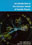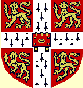© CAMBRIDGE UNIVERSITY PRESS 1998

1.6 More Quarks

``Strange'' mesons and baryons were discovered in the late 1940s, soon after the discovery of the pions. It is apparent that as well as the u and d quarks there exists a so-called strange quark s, and strange particles contain one or more s quarks. An s quark can replace a u or d quark in any baryon or meson to make the strange baryons and strange mesons. The electric charges show that the s quark, like the d, has charge -e/3, and the spectra can be understood if the s is assigned isospin I = 0.

The lowest mass strange mesons are the I = 1/2 doublet, K- (s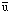, mass 494 MeV) and K0 (d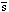, mass 498 MeV). Their antiparticles make up another doublet, the K+(u) and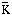0(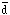s).

The effect of quark replacement on the meson spectrum is illustrated in Fig. 1.4. Each level in the spectrum of Fig. 1.4(b) has a member (d) with charge -e. Fig. 1.4(c) shows the spectrum of strange (s) mesons. There is a correspondence in angular momentum and parity between states in the two spectra. The energy differences are a consequence of the s quark having a much larger mass, of the order of 200 MeV.

The excess of mass of the s quark over the u and d quarks makes the s quark in any strange particle unstable to decay by the weak interaction.

Besides the u, d and s quarks there are considerably heavier quarks: the charmed quark c (mass1.3 GeV / c2, charge 2e/3), the bottom quark b (mass4.3 GeV / c2, charge -e/3), and the top quark t (mass180 GeV / c, charge 2e/3). The quark masses are most remarkable, being even more disparate than the lepton masses. The experimental investigation of the elusive top quark is still in its infancy, but it seems that three quarks of any of the six known flavours can be bound to form a system of states of a baryon (or three antiquarks to form antibaryon states), and any quark-antiquark pair can bind into mesonic states.

The c and b quarks were discovered in e+e- colliding beam machines. Very prominent narrow resonances were observed in the e+e- annihilation cross-sections. Their widths, of less than 15 MeV, distinguished the meson states responsible from those made up of u, d or s quarks. There are two groups of resonant states. The group at around 3 GeV centre of mass energy are known as J /resonances, and are interpreted as charmonium c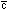states. Another group around 10 GeV, the(upsilon) resonances, are interpreted as bottomonium b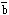states. The current state of knowledge of the cand benergy levels is displayed in Fig. 1.5. We shall discuss these systems in Chapter 17.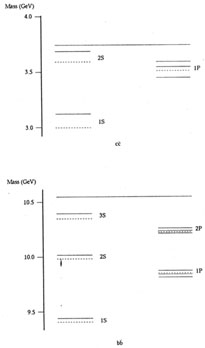Figure 1.5. Energy-level diagrams for charmonium cand bottomonium bstates, below the threshold at which they can decay through the strong interaction to meson pairs (for example c-> c+ u). States labeled 1S, 2S, 3S have orbital angular momentum L = 0 and the 1P, 2P states have L = 1. The intrinsic quark spins can couple to S = 0 to give states with total angular momentum J = L. These states are denoted by - - - -; experimentally they are difficult to detect. The intrinsic quark spins can also couple to give S = 1. States with S = 1 are denoted by ____. Spin-orbit coupling splits the P states with S = 1 to give rise to states with Jp = 0+, 1+, 2+. This spin-orbit splitting is apparent in the figure. All the S = 1 states shown have been measured.

The existence of the top quark was established in 1995 at Fermilab, inp collisions.